GeeksforGeeks App
Open AppBrowser
Continue

# Basic Operations on Binary Tree with Implementations

The tree is a hierarchical Data Structure. A binary tree is a tree that has at most two children. The node which is on the left of the Binary Tree is called “Left-Child” and the node which is the right is called “Right-Child”. Also, the smaller tree or the subtree in the left of the root node is called the “Left sub-tree” and that is on the right is called “Right sub-tree”.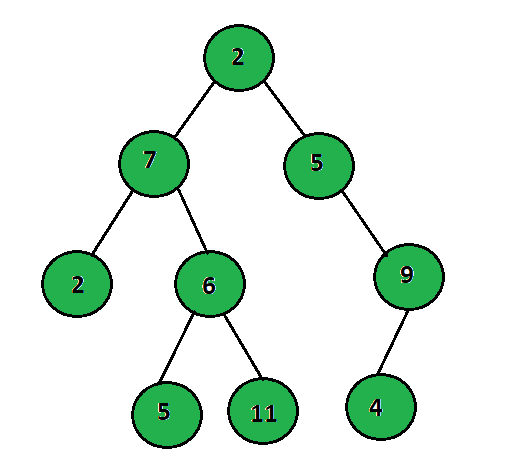Below are the various operations that can be performed on a Binary Tree:

### Creation of Binary Tree:

The idea is to first create the root node of the given tree, then recursively create the left and the right child for each parent node. Below is the program to illustrate the same:

## C++

 `// C++ program to illustrate how to``// create a tree``#include ``using` `namespace` `std;` `// Structure of the Binary Tree``struct` `treenode {``    ``int` `info;``    ``struct` `treenode *left,``        ``*right;``};` `// Function to create the Binary Tree``struct` `treenode* create()``{``    ``int` `data;``    ``struct` `treenode* tree;` `    ``// Dynamically allocating memory``    ``// for the tree-node``    ``tree = ``new` `treenode;` `    ``cout << ``"\nEnter data to be inserted "``         ``<< ``"or type -1 for no insertion : "``;` `    ``// Input from the user``    ``cin >> data;` `    ``// Termination Condition``    ``if` `(data == -1)``        ``return` `0;` `    ``// Assign value from user into tree``    ``tree->info = data;` `    ``// Recursively Call to create the``    ``// left and the right sub tree``    ``cout << ``"Enter left child of : "``         ``<< data;``    ``tree->left = create();` `    ``cout << ``"Enter right child of : "``         ``<< data;``    ``tree->right = create();` `    ``// Return the created Tree``    ``return` `tree;``};` `// Function to perform the inorder``// traversal of the given Tree``void` `inorder(``struct` `treenode* root)``{``    ``// If root is NULL``    ``if` `(root == NULL)``        ``return``;` `    ``// Recursively call for the left``    ``// and the right subtree``    ``inorder(root->left);``    ``cout << root->info << ``"  "``;``    ``inorder(root->right);``}` `// Driver Code``int` `main()``{``    ``// Root Node``    ``struct` `treenode* root = NULL;` `    ``// Function Call``    ``root = create();` `    ``// Perform Inorder Traversal``    ``inorder(root);` `    ``return` `0;``}` `/* Will be creating tree:``                ``2 ``           ``/     \ ``          ``7       5 ``         ``/  \       \ ``        ``2    6       9`` ``*/`

## Java

 `import` `java.util.Scanner;``// class of the Binary Tree node` `class` `TreeNode {``    ``int` `info;``    ``TreeNode left, right;` `    ``TreeNode(``int` `data) {``        ``this``.info = data;``        ``left = right = ``null``;``    ``}``}` `class` `GFG {``    ``static` `Scanner sc = ``new` `Scanner(System.in);``// Function to create the Binary Tree` `    ``static` `TreeNode create() {``        ``int` `data;``        ``System.out.print(``"\nEnter data to be inserted or type -1 for no insertion : "``);``        ``data = sc.nextInt();``        ``if` `(data == -``1``) {``            ``return` `null``;``        ``}``        ``TreeNode tree = ``new` `TreeNode(data);``        ``System.out.print(``"Enter left child of : "` `+ data);``        ``tree.left = create();``        ``System.out.print(``"Enter right child of : "` `+ data);``        ``tree.right = create();``        ``return` `tree;``    ``}``    ``// Perform Inorder Traversal` `    ``static` `void` `inorder(TreeNode root) {``        ``if` `(root == ``null``) {``            ``return``;``        ``}``        ``inorder(root.left);``        ``System.out.print(root.info + ``" "``);``        ``inorder(root.right);``    ``}``// Driver Code` `    ``public` `static` `void` `main(String[] args) {``        ``TreeNode root = ``null``;``        ``root = create();``        ``inorder(root);``    ``}``}`

## Python3

 `#Python equivalent` `# Class of the Binary Tree node``class` `TreeNode:``    ``def` `__init__(``self``, data):``        ``self``.info ``=` `data``        ``self``.left ``=` `None``        ``self``.right ``=` `None` `# Function to create the Binary Tree``def` `create():``    ``data ``=` `int``(``input``(``"\nEnter data to be inserted or type -1 for no insertion : "``))``    ``if` `data ``=``=` `-``1``:``        ``return` `None``    ``tree ``=` `TreeNode(data)``    ``print``(``"Enter left child of : "` `+` `str``(data))``    ``tree.left ``=` `create()``    ``print``(``"Enter right child of : "` `+` `str``(data))``    ``tree.right ``=` `create()``    ``return` `tree` `# Perform Inorder Traversal``def` `inorder(root):``    ``if` `root ``=``=` `None``:``        ``return``    ``inorder(root.left)``    ``print``(root.info, end``=``" "``)``    ``inorder(root.right)` `# Driver Code``if` `__name__ ``=``=` `'__main__'``:``    ``root ``=` `None``    ``root ``=` `create()``    ``inorder(root)`

## C#

 `// C# program to illustrate how to``// create a tree``using` `System;` `// Structure of the Binary Tree``public` `class` `treenode {``    ``public` `int` `info;``    ``public` `treenode left, right;` `    ``public` `treenode()``    ``{``        ``info = 0;``        ``left = ``null``;``        ``right = ``null``;``    ``}``}` `// Class to perform the operations``public` `class` `GFG {``    ``// Function to create the Binary Tree``    ``public` `static` `treenode create()``    ``{``        ``int` `data;``        ``treenode tree = ``new` `treenode();` `        ``Console.WriteLine(``            ``"\nEnter data to be inserted "``            ``+ ``"or type -1 for no insertion : "``);` `        ``// Input from the user``        ``data = Convert.ToInt32(Console.ReadLine());` `        ``// Termination Condition``        ``if` `(data == -1)``            ``return` `null``;` `        ``// Assign value from user into tree``        ``tree.info = data;` `        ``// Recursively Call to create the``        ``// left and the right sub tree``        ``Console.WriteLine(``"Enter left child of : "` `+ data);``        ``tree.left = create();` `        ``Console.WriteLine(``"Enter right child of : "` `+ data);``        ``tree.right = create();` `        ``// Return the created Tree``        ``return` `tree;``    ``}` `    ``// Function to perform the inorder``    ``// traversal of the given Tree``    ``public` `static` `void` `inorder(treenode root)``    ``{``        ``// If root is NULL``        ``if` `(root == ``null``)``            ``return``;` `        ``// Recursively call for the left``        ``// and the right subtree``        ``inorder(root.left);``        ``Console.Write(root.info + ``"  "``);``        ``inorder(root.right);``    ``}` `    ``// Driver Code``    ``public` `static` `void` `Main()``    ``{``        ``// Root Node``        ``treenode root = ``null``;` `        ``// Function Call``        ``root = create();` `        ``// Perform Inorder Traversal``        ``inorder(root);``    ``}``}` `/* Will be creating tree:``                ``2``           ``/     \``          ``7       5``         ``/  \       \``        ``2    6       9`` ``*/`

## Javascript

 `// javascript program to illustrate how to``// create a tree` `// Structure of the Binary Tree``class treenode {``    ` `    ``constructor(){``        ``this``.info = 0;``        ``this``.left = ``null``;``        ``this``.right = ``null``;``    ``}``}` `// Function to create the Binary Tree``function` `create()``{``    ``let data;``    ``let tree = ``new` `treenode();`   `    ``// Input from the user``    ``// data = readInt("\n Enter data to be inserted or type -1 for no insertion :");``    ``data = prompt(``"\n Enter data to be inserted or type -1 for no insertion :"``);`  `    ``// Termination Condition``    ``if` `(data == -1)``        ``return` `0;` `    ``// Assign value from user into tree``    ``tree.info = data;` `    ``// Recursively Call to create the``    ``// left and the right sub tree``    ``console.log(``"Enter left child of : "` `+ data);``    ``tree.left = create();` `    ``console.log(``"Enter right child of : "` `+ data);``    ``tree.right = create();` `    ``// Return the created Tree``    ``return` `tree;``}` `// Function to perform the inorder``// traversal of the given Tree``function` `inorder(root)``{``    ``// If root is NULL``    ``if` `(root == ``null``)``        ``return``;` `    ``// Recursively call for the left``    ``// and the right subtree``    ``inorder(root.left);``    ``console.log(root.info);``    ``inorder(root.right);``}` `// Driver Code``// Root Node``let root = ``null``;` `// Function Call``root = create();` `// Perform Inorder Traversal``inorder(root);` `/* Will be creating tree:``                ``2 ``           ``/     \ ``          ``7       5 ``         ``/  \       \ ``        ``2    6       9`` ``*/` `// The code is contributed by Nidhi goel.`

Output: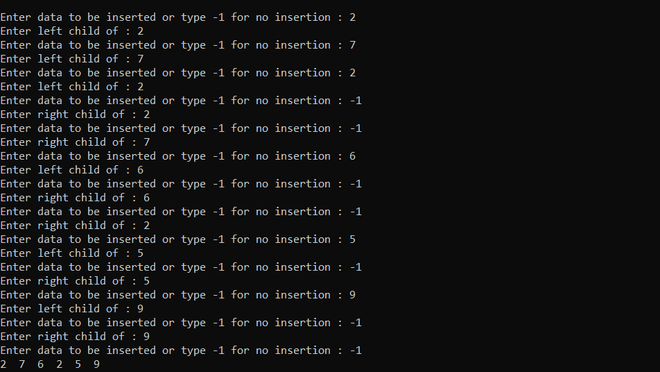Time Complexity: O(N)
Auxiliary Space: O(1)

### Pre-order Traversal:

In this traversal, the root is visited first followed by the left and the right subtree. Below is the program to illustrate the same:

## C++

 `// C++ program to demonstrate the``// pre-order traversal``#include "bits/stdc++.h"``using` `namespace` `std;` `// Structure of the Binary Tree``struct` `treenode {``    ``int` `info;``    ``struct` `treenode *left,``        ``*right;``};` `// Function to create the Binary Tree``struct` `treenode* create()``{``    ``int` `data;``    ``struct` `treenode* tree;` `    ``// Dynamically allocating memory``    ``// for the tree-node``    ``tree = ``new` `treenode;` `    ``cout << ``"\nEnter data to be inserted "``         ``<< ``"or type -1 for no insertion : "``;` `    ``// Input from the user``    ``cin >> data;` `    ``// Termination Condition``    ``if` `(data == -1)``        ``return` `0;` `    ``// Assign value from user into tree``    ``tree->info = data;` `    ``// Recursively Call to create the``    ``// left and the right sub tree``    ``cout << ``"Enter left child of : "``         ``<< data;``    ``tree->left = create();` `    ``cout << ``"Enter right child of : "``         ``<< data;``    ``tree->right = create();` `    ``// Return the created Tree``    ``return` `tree;``};` `// Function to perform the pre-order``// traversal for the given tree``void` `preorder(``struct` `treenode* root)``{``    ``// If the root is NULL``    ``if` `(root == NULL)``        ``return``;` `    ``// Using tree-node type stack STL``    ``stack s;` `    ``while` `((root != NULL) || (!s.empty())) {``        ``if` `(root != NULL) {``            ``// Print the root``            ``cout << root->info << ``" "``;` `            ``// Push the node in the stack``            ``s.push(root);` `            ``// Move to left subtree``            ``root = root->left;``        ``}``        ``else` `{``            ``// Remove the top of stack``            ``root = s.top();``            ``s.pop();``            ``root = root->right;``        ``}``    ``}` `    ``cout << endl;``}` `// Driver Code``int` `main()``{``    ``// Root Node``    ``struct` `treenode* root = NULL;` `    ``// Function Call``    ``root = create();` `    ``// Perform Inorder Traversal``    ``preorder(root);` `    ``return` `0;``}` `/* Will be creating tree:``                ``2 ``           ``/     \ ``          ``7       5 ``         ``/  \       \ ``        ``2    6       9`` ``*/`

## Python3

 `# Python code addition` `# Structure of the Binary Tree``class` `TreeNode:``    ``def` `__init__(``self``, val``=``0``, left``=``None``, right``=``None``):``        ``self``.val ``=` `val``        ``self``.left ``=` `left``        ``self``.right ``=` `right` `# Function to create the Binary Tree``def` `create():``    ``data ``=` `int``(``input``(``"\nEnter data to be inserted or type -1 for no insertion: "``))` `    ``# Termination Condition``    ``if` `data ``=``=` `-``1``:``        ``return` `None` `    ``# Assign value from user into tree``    ``root ``=` `TreeNode(data)` `    ``# Recursively Call to create the left and the right sub tree``    ``print``(``"Enter left child of:"``, data)``    ``root.left ``=` `create()` `    ``print``(``"Enter right child of:"``, data)``    ``root.right ``=` `create()` `    ``# Return the created Tree``    ``return` `root` `# Function to perform the pre-order traversal for the given tree``def` `preorder(root):``    ``# If the root is None``    ``if` `not` `root:``        ``return``    ` `    ``# Using tree-node type stack STL``    ``stack ``=` `[]` `    ``while` `root ``or` `stack:``        ``if` `root:``            ``# Print the root``            ``print``(root.val, end``=``" "``)` `            ``# Push the node in the stack``            ``stack.append(root)` `            ``# Move to left subtree``            ``root ``=` `root.left``        ``else``:``            ``# Remove the top of stack``            ``root ``=` `stack.pop()``            ``root ``=` `root.right` `    ``print``()` `# Driver Code``if` `__name__ ``=``=` `"__main__"``:``    ``# Root Node``    ``root ``=` `None` `    ``# Function Call``    ``root ``=` `create()` `    ``# Perform Pre-order Traversal``    ``preorder(root)` `# The code is contributed by Nidhi goel.`

## Javascript

 `class TreeNode {``  ``constructor(info) {``    ``this``.info = info;``    ``this``.left = ``null``;``    ``this``.right = ``null``;``  ``}``}` `function` `create() {``  ``let data = prompt(``    ``"Enter data to be inserted or type -1 for no insertion : "``  ``);` `  ``// Termination Condition``  ``if` `(data == -1) ``return` `null``;` `  ``// Assign value from user into tree``  ``let tree = ``new` `TreeNode(data);` `  ``// Recursively Call to create the``  ``// left and the right sub tree``  ``tree.left = create();``  ``tree.right = create();` `  ``// Return the created Tree``  ``return` `tree;``}` `// Function to perform the pre-order``// traversal for the given tree``function` `preorder(root) {``  ``// If the root is NULL``  ``if` `(root == ``null``) ``return``;` `  ``// Using tree-node type stack STL``  ``let s = [];` `  ``while` `(root != ``null` `|| s.length > 0) {``    ``if` `(root != ``null``) {``      ``// Print the root``      ``console.log(root.info);` `      ``// Push the node in the stack``      ``s.push(root);` `      ``// Move to left subtree``      ``root = root.left;``    ``} ``else` `{``      ``// Remove the top of stack``      ``root = s.pop();``      ``root = root.right;``    ``}``  ``}``}` `// Driver Code` `// Root Node``let root = ``null``;` `// Function Call``root = create();` `// Perform Inorder Traversal``preorder(root);`

Output: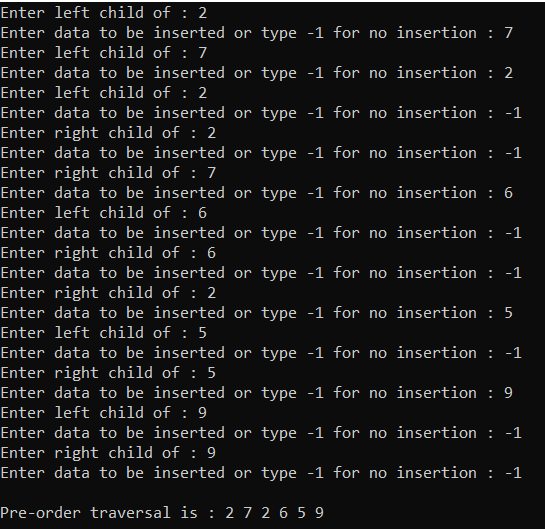Time Complexity: O(N)
Auxiliary Space: O(N)

### In-order Traversal:

In this traversal, the left subtree is visited first followed by the root and the right subtree. Below is the program to illustrate the same:

## C++

 `// C++ program to illustrate how to``// create a tree``#include "bits/stdc++.h"``using` `namespace` `std;` `// Structure of the Binary Tree``struct` `treenode {``    ``int` `info;``    ``struct` `treenode *left,``        ``*right;``};` `// Function to create the Binary Tree``struct` `treenode* create()``{``    ``int` `data;``    ``struct` `treenode* tree;` `    ``// Dynamically allocating memory``    ``// for the tree-node``    ``tree = ``new` `treenode;` `    ``cout << ``"\nEnter data to be inserted "``         ``<< ``"or type -1 for no insertion : "``;` `    ``// Input from the user``    ``cin >> data;` `    ``// Termination Condition``    ``if` `(data == -1)``        ``return` `0;` `    ``// Assign value from user into tree``    ``tree->info = data;` `    ``// Recursively Call to create the``    ``// left and the right sub tree``    ``cout << ``"Enter left child of : "``         ``<< data;``    ``tree->left = create();` `    ``cout << ``"Enter right child of : "``         ``<< data;``    ``tree->right = create();` `    ``// Return the created Tree``    ``return` `tree;``};` `// Function to perform the inorder``// traversal of the given Tree``void` `inorder(``struct` `treenode* root)``{``    ``// If root is NULL``    ``if` `(root == NULL)``        ``return``;` `    ``// Recursively call for the left``    ``// and the right subtree``    ``inorder(root->left);``    ``cout << root->info << ``"  "``;``    ``inorder(root->right);``}` `// Driver Code``int` `main()``{``    ``// Root Node``    ``struct` `treenode* root = NULL;` `    ``// Function Call``    ``root = create();` `    ``// Perform Inorder Traversal``    ``inorder(root);` `    ``return` `0;``}` `/* Will be creating tree:``                ``2 ``           ``/     \ ``          ``7       5 ``         ``/  \       \ ``        ``2    6       9`` ``*/`

## Javascript

 `// JavaScript program to illustrate how to``// create a tree` `// Structure of the Binary Tree``class TreeNode {``    ``constructor(info) {``        ``this``.info = info;``        ``this``.left = ``null``;``        ``this``.right = ``null``;``    ``}``}` `// Function to create the Binary Tree``function` `create() {``    ``const tree = ``new` `TreeNode();` `    ``let data;``    ``console.log(``"Enter data to be inserted or type -1 for no insertion : "``);` `    ``// Input from the user``    ``data = parseInt(prompt());` `    ``// Termination Condition``    ``if` `(data === -1)``        ``return` `null``;` `    ``// Assign value from user into tree``    ``tree.info = data;` `    ``// Recursively Call to create the``    ``// left and the right sub tree``    ``console.log(`Enter left child of \${data}: `);``    ``tree.left = create();` `    ``console.log(`Enter right child of \${data}: `);``    ``tree.right = create();` `    ``// Return the created Tree``    ``return` `tree;``}` `// Function to perform the inorder``// traversal of the given Tree``function` `inorder(root) {``    ``// If root is NULL``    ``if` `(root == ``null``)``        ``return``;` `    ``// Recursively call for the left``    ``// and the right subtree``    ``inorder(root.left);``    ``console.log(`\${root.info} `);``    ``inorder(root.right);``}` `// Driver Code``function` `main() {``    ``// Root Node``    ``let root = ``null``;` `    ``// Function Call``    ``root = create();` `    ``// Perform Inorder Traversal``    ``inorder(root);``}` `// Invoke the main function``main();` `/* Will be creating tree:``             ``2 ``           ``/   \ ``          ``7     5 ``         ``/  \     \ ``        ``2    6     9`` ``*/`

Output: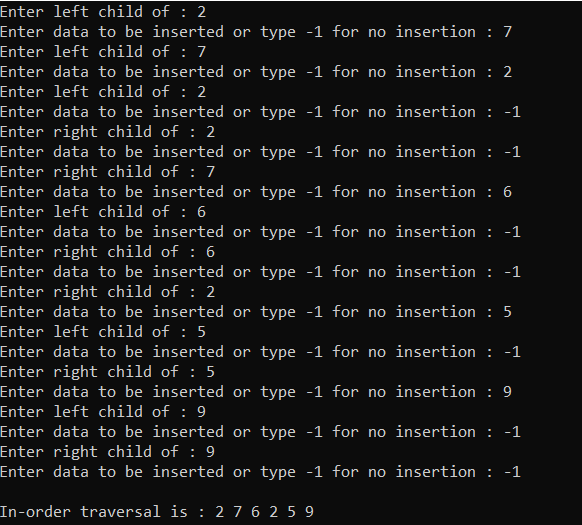Time Complexity: O(N)
Auxiliary Space: O(N)

### Post-order Traversal:

In this traversal, the left subtree is visited first, followed by the right subtree and root node. Below is the program to illustrate the same:

## C++

 `// C++ program to implement the``// post-order traversal``#include "bits/stdc++.h"``using` `namespace` `std;` `// Structure of the Binary Tree``struct` `treenode {``    ``int` `info;``    ``struct` `treenode *left,``        ``*right;``};` `// Function to create the Binary Tree``struct` `treenode* create()``{``    ``int` `data;``    ``struct` `treenode* tree;` `    ``// Dynamically allocating memory``    ``// for the tree-node``    ``tree = ``new` `treenode;` `    ``cout << ``"\nEnter data to be inserted "``         ``<< ``"or type -1 for no insertion : "``;` `    ``// Input from the user``    ``cin >> data;` `    ``// Termination Condition``    ``if` `(data == -1)``        ``return` `0;` `    ``// Assign value from user into tree``    ``tree->info = data;` `    ``// Recursively Call to create the``    ``// left and the right sub tree``    ``cout << ``"Enter left child of : "``         ``<< data;``    ``tree->left = create();` `    ``cout << ``"Enter right child of : "``         ``<< data;``    ``tree->right = create();` `    ``// Return the created Tree``    ``return` `tree;``};` `// Function to perform the post-order``// traversal of the given tree``void` `postorder(``struct` `treenode* root)``{``    ``// If the root is NULL``    ``return``;` `    ``stack s3;``    ``struct` `treenode* previous = NULL;` `    ``do` `{``        ``// Iterate until root is present``        ``while` `(root != NULL) {``            ``s3.push(root);``            ``root = root->left;``        ``}` `        ``while` `(root == NULL && (!s3.empty())) {``            ``root = s3.top();` `            ``// If the right subtree is NULL``            ``if` `(root->right == NULL``                ``|| root->right == previous) {``                ``// Print the root information``                ``cout << root->info << ``" "``;``                ``s3.pop();` `                ``// Update the previous``                ``previous = root;``                ``root = NULL;``            ``}` `            ``// Otherwise``            ``else``                ``root = root->right;``        ``}` `    ``} ``while` `(!s3.empty());``    ``cout << endl;``}` `// Driver Code``int` `main()``{``    ``// Root Node``    ``struct` `treenode* root = NULL;` `    ``// Function Call``    ``root = create();` `    ``// Perform Inorder Traversal``    ``postorder(root);` `    ``return` `0;``}` `/* Will be creating tree:``                ``2 ``           ``/     \ ``          ``7       5 ``         ``/  \       \ ``        ``2    6       9`` ``*/`

Output: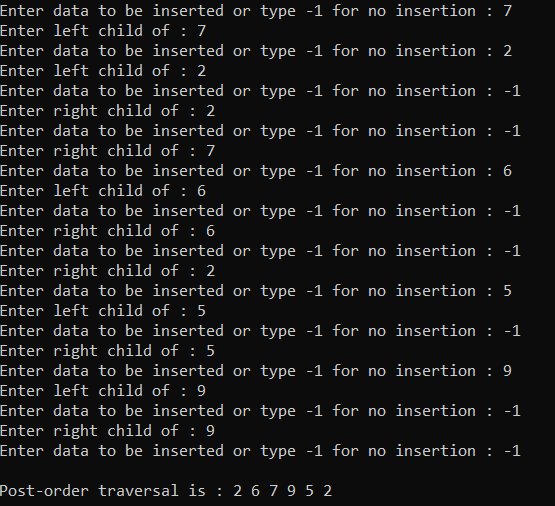Time Complexity: O(N)
Auxiliary Space: O(N)

### Level-order Traversal:

In this traversal, the given tree is traversal level-wise. Below is the program to illustrate the same:

## C++

 `// C++ program to illustrate the``// level order traversal#include "bits/stdc++.h"``using` `namespace` `std;` `// Structure of the Binary Tree``struct` `treenode {``    ``int` `info;``    ``struct` `treenode *left,``        ``*right;``};` `// Function to create the Binary Tree``struct` `treenode* create()``{``    ``int` `data;``    ``struct` `treenode* tree;` `    ``// Dynamically allocating memory``    ``// for the tree-node``    ``tree = ``new` `treenode;` `    ``cout << ``"\nEnter data to be inserted "``         ``<< ``"or type -1 for no insertion : "``;` `    ``// Input from the user``    ``cin >> data;` `    ``// Termination Condition``    ``if` `(data == -1)``        ``return` `0;` `    ``// Assign value from user into tree``    ``tree->info = data;` `    ``// Recursively Call to create the``    ``// left and the right sub tree``    ``cout << ``"Enter left child of : "``         ``<< data;``    ``tree->left = create();` `    ``cout << ``"Enter right child of : "``         ``<< data;``    ``tree->right = create();` `    ``// Return the created Tree``    ``return` `tree;``};` `// Function to perform the level-order``// traversal``void` `levelorder(``struct` `treenode* root)``{``    ``// If the root is NULL``    ``if` `(root == NULL)``        ``return``;` `    ``// Use queue for traversal``    ``queue q;` `    ``// Print the root's value and``    ``// push it into the queue``    ``cout << root->info << ``" "``;``    ``q.push(root);` `    ``// Iterate until queue is non-empty``    ``while` `(!q.empty()) {``        ``// Get the front node``        ``root = q.front();``        ``q.pop();` `        ``// If the root has the left child``        ``if` `(root->left) {``            ``cout << root->left->info``                 ``<< ``" "``;``            ``q.push(root->left);``        ``}` `        ``// If the root has the right child``        ``if` `(root->right) {``            ``cout << root->right->info``                 ``<< ``" "``;``            ``q.push(root->right);``        ``}``    ``}``    ``cout << endl;``}` `// Driver Code``int` `main()``{``    ``// Root Node``    ``struct` `treenode* root = NULL;` `    ``// Function Call``    ``root = create();` `    ``// Perform Inorder Traversal``    ``levelorder(root);` `    ``return` `0;``}` `/* Will be creating tree:``                ``2 ``           ``/     \ ``          ``7       5 ``         ``/  \       \ ``        ``2    6       9`` ``*/`

Output: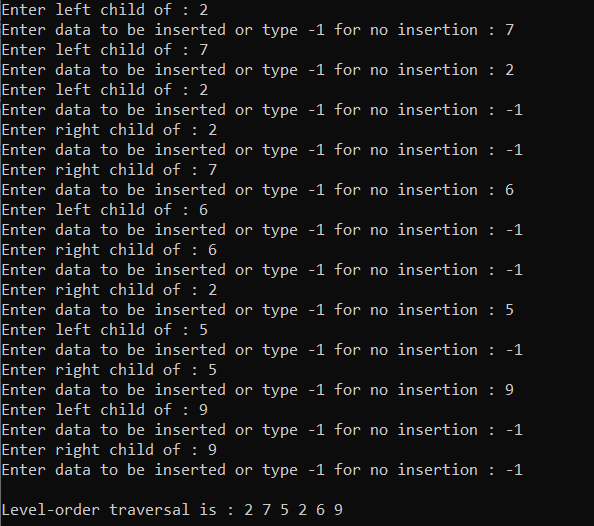Time Complexity: O(N)
Auxiliary Space: O(N)

### The maximum element of the Binary Tree:

The element which is largest among all the elements of the binary tree is called the maximum element. Below is the program to illustrate the same:

## C++

 `// C++ program for the above approach``#include "bits/stdc++.h"``using` `namespace` `std;` `// Structure of the Binary Tree``struct` `treenode {``    ``int` `info;``    ``struct` `treenode *left, *right;``};` `// Function to create the Binary Tree``struct` `treenode* create()``{``    ``int` `data;``    ``struct` `treenode* tree;` `    ``// Dynamically allocating memory``    ``// for the tree-node``    ``tree = ``new` `treenode;` `    ``cout << ``"\nEnter data to be inserted "``         ``<< ``"or type -1 for no insertion : "``;` `    ``// Input from the user``    ``cin >> data;` `    ``// Termination Condition``    ``if` `(data == -1)``        ``return` `0;` `    ``// Assign value from user into tree``    ``tree->info = data;` `    ``// Recursively Call to create the``    ``// left and the right sub tree``    ``cout << ``"Enter left child of : "` `<< data;``    ``tree->left = create();` `    ``cout << ``"Enter right child of : "` `<< data;``    ``tree->right = create();` `    ``// Return the created Tree``    ``return` `tree;``};` `// Function to find the maximum element``// in the given Binary Tree``int` `FindMax(``struct` `treenode* root)``{``    ``// If the tree is empty``    ``if` `(root == NULL)``        ``return` `0;` `    ``queue q;``    ``int` `max;``    ``struct` `treenode* temp;` `    ``max = root->info;` `    ``// Push the root in the queue``    ``q.push(root);` `    ``// Iterate until queue is non-empty``    ``while` `(!q.empty()) {` `        ``// Get the front node of``        ``// the tree``        ``root = q.front();``        ``temp = root;``        ``q.pop();` `        ``// Update the maximum value``        ``// of the Tree``        ``if` `(max < temp->info)``            ``max = temp->info;` `        ``if` `(root->left) {``            ``q.push(root->left);``        ``}``        ``if` `(root->right) {``            ``q.push(root->right);``        ``}``    ``}` `    ``// Return the maximum value``    ``return` `max;``}` `// Driver Code``int` `main()``{``    ``// Root Node``    ``struct` `treenode* root = NULL;` `    ``// Function Call``    ``root = create();` `    ``// Perform Inorder Traversal``    ``FindMax(root);` `    ``return` `0;``}` `/* Will be creating tree:``                ``2 ``           ``/     \ ``          ``7       5 ``         ``/  \       \ ``        ``2    6       9`` ``*/`

Output: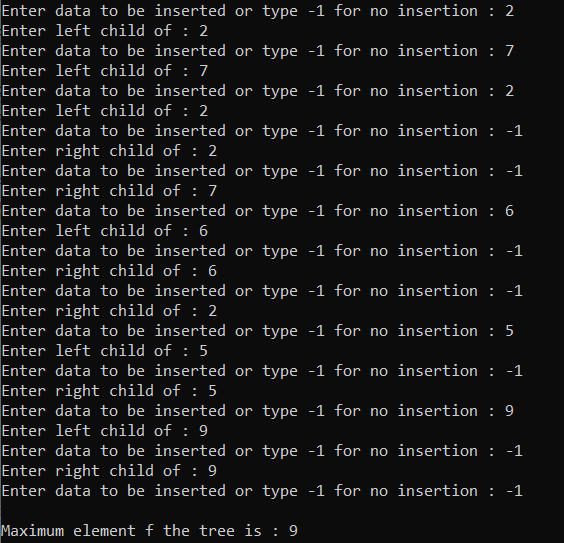Time Complexity: O(N)
Auxiliary Space: O(N)

### Search for an element:

The approach to search for any particular element in the tree node is to perform any tree traversal on the given tree and check if there exists any node with the given searched value or not. If found to be true, then print “Element is Found”. Otherwise, print “Element  Not  Found”

Below is the program to illustrate the same:

## C++

 `// C++ program for the above approach``#include "bits/stdc++.h"``using` `namespace` `std;` `// Structure of the Binary Tree``struct` `treenode {``    ``int` `info;``    ``struct` `treenode *left, *right;``};` `// Function to create the Binary Tree``struct` `treenode* create()``{``    ``int` `data;``    ``struct` `treenode* tree;` `    ``// Dynamically allocating memory``    ``// for the tree-node``    ``tree = ``new` `treenode;` `    ``cout << ``"\nEnter data to be inserted "``         ``<< ``"or type -1 for no insertion : "``;` `    ``// Input from the user``    ``cin >> data;` `    ``// Termination Condition``    ``if` `(data == -1)``        ``return` `0;` `    ``// Assign value from user into tree``    ``tree->info = data;` `    ``// Recursively Call to create the``    ``// left and the right sub tree``    ``cout << ``"Enter left child of : "` `<< data;``    ``tree->left = create();` `    ``cout << ``"Enter right child of : "` `<< data;``    ``tree->right = create();` `    ``// Return the created Tree``    ``return` `tree;``};` `// Function to search an element in the``// given Binary Tree``int` `FindElement(``struct` `treenode* root,``                ``int` `data)``{``    ``// If the root is NULL``    ``if` `(root == NULL)``        ``return` `0;` `    ``queue q;``    ``struct` `treenode* temp;``    ``if` `(!root)``        ``return` `0;` `    ``else` `{``        ``// Push the root``        ``q.push(root);` `        ``// Perform the level-order traversal``        ``while` `(!q.empty()) {``            ``// Get the root``            ``root = q.front();``            ``temp = root;``            ``q.pop();` `            ``// If the node with value data``            ``// exists then return 1``            ``if` `(data == temp->info)``                ``return` `1;` `            ``// Recursively push the left and``            ``// the right child of the node``            ``if` `(root->left) {``                ``q.push(root->left);``            ``}``            ``if` `(root->right) {``                ``q.push(root->right);``            ``}``        ``}` `        ``// Otherwise, not found``        ``return` `0;``    ``}``}` `// Driver Code``int` `main()``{``    ``int` `data;` `    ``// Root of the tree``    ``struct` `treenode* root = NULL;` `    ``// Create the Tree``    ``root = create();` `    ``cout << ``"\nEnter element to searched : "``;``    ``cin >> data;` `    ``// Function Call``    ``if` `(FindElement(root, data) == 1)``        ``cout << ``"\nElement is found"``;``    ``else``        ``cout << ``"Element is not found"``;``    ``return` `0;``}` `/* Will be creating tree:``                ``2``           ``/     \``          ``7       5``         ``/  \       \``        ``2    6       9`` ``*/`

Output: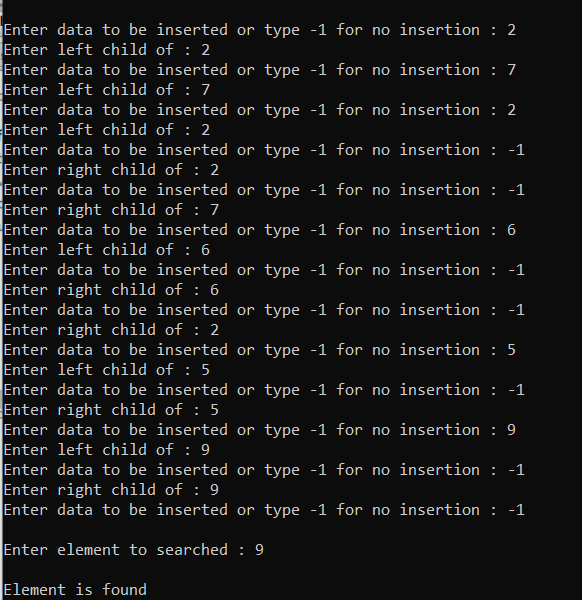Time Complexity: O(log N)
Auxiliary Space: O(N)

### Reverse Level Order Traversal:

Below is the program to illustrate the same:

## C++

 `// C++ program for the above approach``#include "bits/stdc++.h"``using` `namespace` `std;` `// Structure of the Binary Tree``struct` `treenode {``    ``int` `info;``    ``struct` `treenode *left, *right;``};` `// Function to create the Binary Tree``struct` `treenode* create()``{``    ``int` `data;``    ``struct` `treenode* tree;` `    ``// Dynamically allocating memory``    ``// for the tree-node``    ``tree = ``new` `treenode;` `    ``cout << ``"\nEnter data to be inserted "``         ``<< ``"or type -1 for no insertion : "``;` `    ``// Input from the user``    ``cin >> data;` `    ``// Termination Condition``    ``if` `(data == -1)``        ``return` `0;` `    ``// Assign value from user into tree``    ``tree->info = data;` `    ``// Recursively Call to create the``    ``// left and the right sub tree``    ``cout << ``"Enter left child of : "` `<< data;``    ``tree->left = create();` `    ``cout << ``"Enter right child of : "` `<< data;``    ``tree->right = create();` `    ``// Return the created Tree``    ``return` `tree;``};` `// Function to print the reverse level``// order traversal of the given tree``void` `reversetree(``struct` `treenode* root)``{``    ``// If the root is NULL``    ``if` `(root == NULL)``        ``return``;` `    ``queue q;``    ``stack<``int``> s;``    ``struct` `treenode* temp;``    ``q.push(root);` `    ``// Until queue is empty``    ``while` `(!q.empty()) {``        ``// Get the front node``        ``temp = q.front();``        ``q.pop();` `        ``// Push every countered node``        ``// data into stack``        ``s.push(temp->info);` `        ``// Check for the left subtree``        ``if` `(temp->left)``            ``q.push(temp->left);` `        ``// Check for the right subtree``        ``if` `(temp->right)``            ``q.push(temp->right);``    ``}` `    ``// While S is non-empty, print``    ``// all the nodes``    ``while` `(!s.empty()) {``        ``cout << s.top() << ``" "``;``        ``s.pop();``    ``}``}` `// Driver Code``int` `main()``{``    ``// Create root node``    ``struct` `treenode* root = NULL;` `    ``// Create a tree``    ``root = create();` `    ``cout << ``"\nReversed tree is :  "``;``    ``reversetree(root);``    ``return` `0;``}``/* Will be creating tree:``                ``2``           ``/     \``          ``7       5``         ``/  \       \``        ``2    6       9``*/`

Output: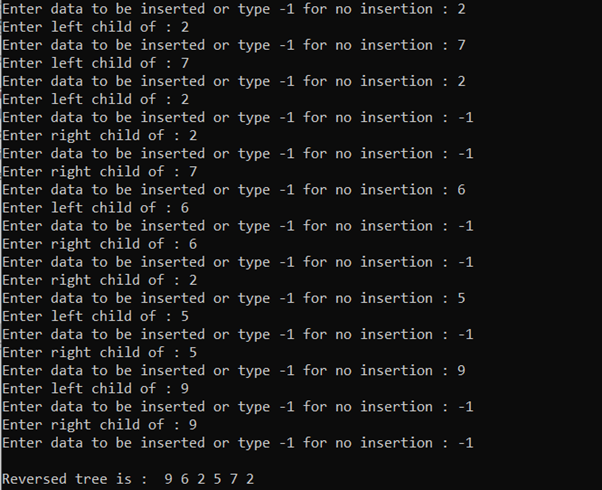Time Complexity: O(N)
Auxiliary Space: O(N)

### Height of the tree:

The height of the binary tree is the longest path from the root node to any leaf node in the tree. Below is the program to illustrate the same:

## C++

 `// C++ program for the above approach``#include "bits/stdc++.h"``using` `namespace` `std;` `// Structure of the Binary Tree``struct` `treenode {``    ``int` `info;``    ``struct` `treenode *left, *right;``};` `// Function to create the Binary Tree``struct` `treenode* create()``{``    ``int` `data;``    ``struct` `treenode* tree;` `    ``// Dynamically allocating memory``    ``// for the tree-node``    ``tree = ``new` `treenode;` `    ``cout << ``"\nEnter data to be inserted "``         ``<< ``"or type -1 for no insertion : "``;` `    ``// Input from the user``    ``cin >> data;` `    ``// Termination Condition``    ``if` `(data == -1)``        ``return` `0;` `    ``// Assign value from user into``    ``// the tree``    ``tree->info = data;` `    ``// Recursively Call to create the``    ``// left and the right sub tree``    ``cout << ``"Enter left child of : "``         ``<< data;``    ``tree->left = create();` `    ``cout << ``"Enter right child of : "``         ``<< data;``    ``tree->right = create();` `    ``// Return the created Tree``    ``return` `tree;``};` `// Function to find the height of``// the given Binary tree``int` `height(``struct` `treenode* root)``{``    ``int` `x, y;` `    ``// If root is NOT NULL``    ``if` `(root != NULL) {``        ``// x will contain the height``        ``// of left subtree``        ``x = height(root->left);` `        ``// y will contain the height``        ``// of right subtree``        ``y = height(root->right);` `        ``if` `(x > y)` `            ``// Leaf node has one height``            ``// so x or y + 1``            ``return` `x + 1;``        ``else``            ``return` `y + 1;``    ``}``    ``return` `0;``}` `// Driver Code``int` `main()``{``    ``// Root Node``    ``struct` `treenode* root = NULL;` `    ``// Create the tree``    ``root = create();` `    ``cout << ``"\nHeight of the tree is :  "``         ``<< height(root);` `    ``return` `0;``}``/* Will be creating tree:``                ``2``           ``/     \``          ``7       5``         ``/  \       \``        ``2    6       9`` ``*/`

Output: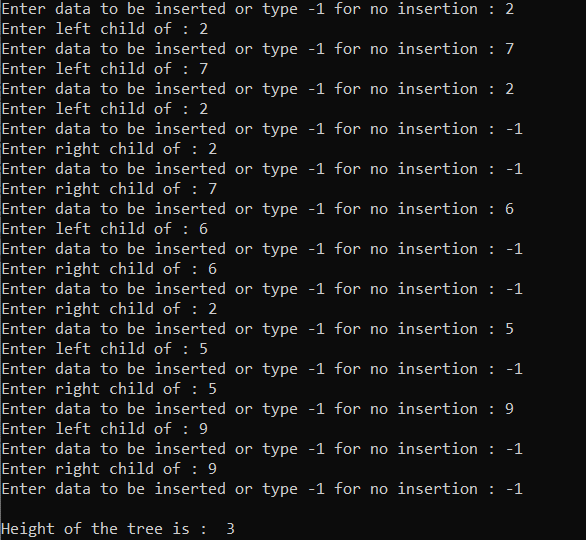Time Complexity: O(N)
Auxiliary Space: O(1)

### The deepest node of the tree:

The node which is present at the maximum or the last level is called the deepest node. Below is the program to implement the above approach:

## C++

 `// C++ program for the above approach``#include "bits/stdc++.h"``using` `namespace` `std;` `// Structure of the Binary Tree``struct` `treenode {``    ``int` `info;``    ``struct` `treenode *left, *right;``};` `// Function to create the Binary Tree``struct` `treenode* create()``{``    ``int` `data;``    ``struct` `treenode* tree;` `    ``// Dynamically allocating memory``    ``// for the tree-node``    ``tree = ``new` `treenode;` `    ``cout << ``"\nEnter data to be inserted "``         ``<< ``"or type -1 for no insertion : "``;` `    ``// Input from the user``    ``cin >> data;` `    ``// Termination Condition``    ``if` `(data == -1)``        ``return` `0;` `    ``// Assign value from user into tree``    ``tree->info = data;` `    ``// Recursively Call to create the``    ``// left and the right sub tree``    ``cout << ``"Enter left child of : "` `<< data;``    ``tree->left = create();` `    ``cout << ``"Enter right child of : "` `<< data;``    ``tree->right = create();` `    ``// Return the created Tree``    ``return` `tree;``};` `// Function to find the deepest node``// of the given Binary Tree``int` `deepest(``struct` `treenode* root)``{``    ``// If the root is NULL``    ``if` `(root == NULL)``        ``return` `0;` `    ``queue q;` `    ``q.push(root);` `    ``// While queue is non-empty``    ``while` `(!q.empty()) {``        ``// Get the front node of queue``        ``root = q.front();``        ``q.pop();` `        ``// Check for the left and``        ``// the right subtree``        ``if` `(root->left)``            ``q.push(root->left);``        ``if` `(root->right)``            ``q.push(root->right);``    ``}` `    ``// Return the value for the``    ``// deepest node``    ``return` `(root->info);``}` `// Driver Code``int` `main()``{``    ``// Root Node``    ``struct` `treenode* root = NULL;` `    ``// Create the tree``    ``root = create();` `    ``cout << ``"\nDeepest node of the tree is :  "` `<< deepest(root);` `    ``return` `0;``}` `/* Will be creating tree:``                ``2``           ``/     \``          ``7       5``         ``/  \       \``        ``2    6       9`` ``*/`

Output: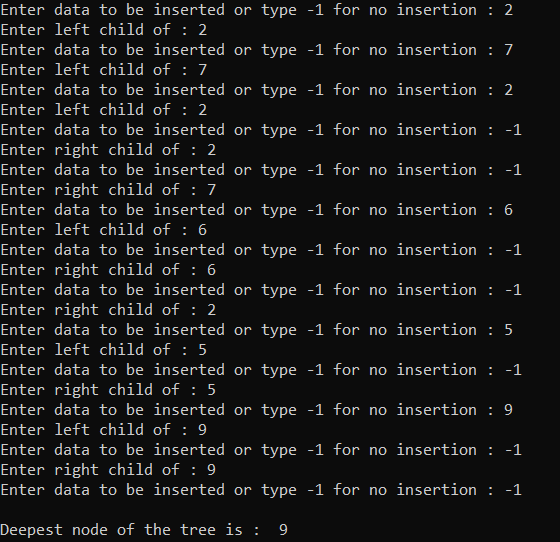Time Complexity: O(N)
Auxiliary Space: O(N)

### Left view of the tree:

Below is the program to implement the same:

## C++

 `// C++ program for the above approach``#include "bits/stdc++.h"``using` `namespace` `std;` `// Structure of the Binary Tree``struct` `treenode {``    ``int` `info;``    ``struct` `treenode *left, *right;``};` `// Function to create the Binary Tree``struct` `treenode* create()``{``    ``int` `data;``    ``struct` `treenode* tree;` `    ``// Dynamically allocating memory``    ``// for the tree-node``    ``tree = ``new` `treenode;` `    ``cout << ``"\nEnter data to be inserted "``         ``<< ``"or type -1 for no insertion : "``;` `    ``// Input from the user``    ``cin >> data;` `    ``// Termination Condition``    ``if` `(data == -1)``        ``return` `0;` `    ``// Assign value from user into tree``    ``tree->info = data;` `    ``// Recursively Call to create the``    ``// left and the right sub tree``    ``cout << ``"Enter left child of : "` `<< data;``    ``tree->left = create();` `    ``cout << ``"Enter right child of : "` `<< data;``    ``tree->right = create();` `    ``// Return the created Tree``    ``return` `tree;``};` `// Stores the maximum left size``int` `maxlevelleft = 0;` `// Function to print the left view of``// the tree``void` `leftview(``struct` `treenode* root,``              ``int` `level)``{``    ``if` `(root == NULL)``        ``return``;` `    ``// If current level is at least``    ``// the maximum left level``    ``if` `(level >= maxlevelleft) {``        ``// Print the data``        ``cout << root->info << ``" "``;``        ``maxlevelleft++;``    ``}` `    ``// Left and Right Subtree``    ``// recursive calls``    ``leftview(root->left, level + 1);``    ``leftview(root->right, level + 1);``}` `// Driver Code``int` `main()``{``    ``// Root Node``    ``struct` `treenode* root = NULL;` `    ``// Create the tree``    ``root = create();` `    ``cout << ``"\nLeft view of the tree is :  "``;` `    ``// Function Call``    ``leftview(root, 0);` `    ``return` `0;``}` `/* Will be creating tree:``                ``2``           ``/     \``          ``7       5``         ``/  \       \``        ``2    6       9`` ``*/`

Output: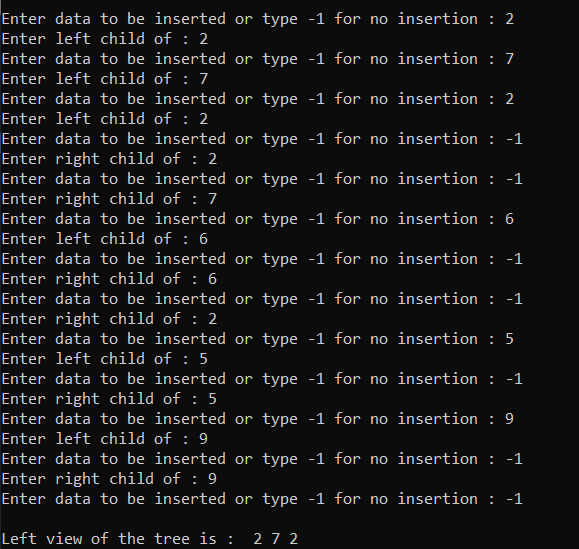Time Complexity: O(N)
Auxiliary Space: O(1)

### Right view of the tree:

Below is the program to illustrate the same:

## C++

 `// C++ program to demonstrate the``// above concepts``#include "bits/stdc++.h"``using` `namespace` `std;` `// Structure of the Binary Tree``struct` `treenode {``    ``int` `info;``    ``struct` `treenode *left,``        ``*right;``};` `// Function to create the Binary Tree``struct` `treenode* create()``{``    ``int` `data;``    ``struct` `treenode* tree;` `    ``// Dynamically allocating memory``    ``// for the tree-node``    ``tree = ``new` `treenode;` `    ``cout << ``"\nEnter data to be inserted "``         ``<< ``"or type -1 for no insertion : "``;` `    ``// Input from the user``    ``cin >> data;` `    ``// Termination Condition``    ``if` `(data == -1)``        ``return` `0;` `    ``// Assign value from user into tree``    ``tree->info = data;` `    ``// Recursively Call to create the``    ``// left and the right sub tree``    ``cout << ``"Enter left child of : "``         ``<< data;``    ``tree->left = create();` `    ``cout << ``"Enter right child of : "``         ``<< data;``    ``tree->right = create();` `    ``// Return the created Tree``    ``return` `tree;``};` `// Stores the maximum right level``int` `maxlevelright = 0;` `// Function to print the right view of``// the given Binary tree``void` `rightview(``struct` `treenode* root,``               ``int` `level)``{``    ``// If the root is NULL``    ``if` `(root == NULL)``        ``return``;` `    ``// If the current level is greater``    ``// than the maximum right level``    ``if` `(level >= maxlevelright) {``        ``// Print the data``        ``cout << root->info << ``" "``;``        ``maxlevelright++;``    ``}` `    ``// Recursively call for the right``    ``// and the left subtree``    ``rightview(root->right, level + 1);``    ``rightview(root->left, level + 1);``}` `// Driver Code``int` `main()``{``    ``// Root Node``    ``struct` `treenode* root = NULL;` `    ``// Create the tree``    ``root = create();` `    ``cout << ``"\nRight view of the tree is :  "``;` `    ``rightview(root, 0);` `    ``return` `0;``}``/* Will be creating tree:``                ``2``           ``/     \``          ``7       5``         ``/  \       \``        ``2    6       9``*/`

Output: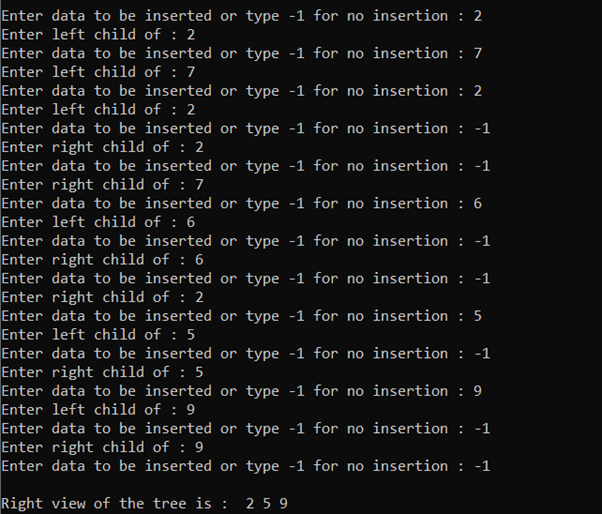Time Complexity: O(N)
Auxiliary Space: O(1)

### Top view of the tree:

Below is the program to illustrate the same:

## C++

 `// C++ program to demonstrate the``// above concepts``#include "bits/stdc++.h"``using` `namespace` `std;` `// Structure of the Binary Tree``struct` `treenode {``    ``int` `info;``    ``struct` `treenode *left,``        ``*right;``};` `// Function to create the Binary Tree``struct` `treenode* create()``{``    ``int` `data;``    ``struct` `treenode* tree;` `    ``// Dynamically allocating memory``    ``// for the tree-node``    ``tree = ``new` `treenode;` `    ``cout << ``"\nEnter data to be inserted "``         ``<< ``"or type -1 for no insertion : "``;` `    ``// Input from the user``    ``cin >> data;` `    ``// Termination Condition``    ``if` `(data == -1)``        ``return` `0;` `    ``// Assign value from user into tree``    ``tree->info = data;` `    ``// Recursively Call to create the``    ``// left and the right sub tree``    ``cout << ``"Enter left child of : "``         ``<< data;``    ``tree->left = create();` `    ``cout << ``"Enter right child of : "``         ``<< data;``    ``tree->right = create();` `    ``// Return the created Tree``    ``return` `tree;``};` `// Initialize an ordered map``map<``int``, ``int``> HashMap;` `// Iterator for the map``map<``int``, ``int``>::iterator it;` `// Function to print the top view``// of the given Binary Tree``void` `topview(``struct` `treenode* root,``             ``int` `level)``{``    ``// If the root is NULL``    ``if` `(root == NULL)``        ``return``;` `    ``// Get the level``    ``int` `i = HashMap.count(level);` `    ``// Update the root information``    ``if` `(i == 0)``        ``HashMap[level] = root->info;` `    ``// Left and Right recursive calls``    ``topview(root->left, level - 1);``    ``topview(root->right, level + 1);` `    ``// Update the current level``    ``// with the root's value``    ``HashMap[level] = root->info;` `    ``return``;``}` `// Driver Code``int` `main()``{``    ``// Root Node``    ``struct` `treenode* root = NULL;` `    ``// Create a tree``    ``root = create();` `    ``topview(root, 0);``    ``cout << ``"\nTop view of the tree is : "``;` `    ``for` `(it = HashMap.begin();``         ``it != HashMap.end(); it++) {``        ``cout << it->second << ``" "``;``    ``}` `    ``return` `0;``}``/* Will be creating tree:``                ``2``           ``/     \``          ``7       5``         ``/  \       \``        ``2    6       9``*/`

Output: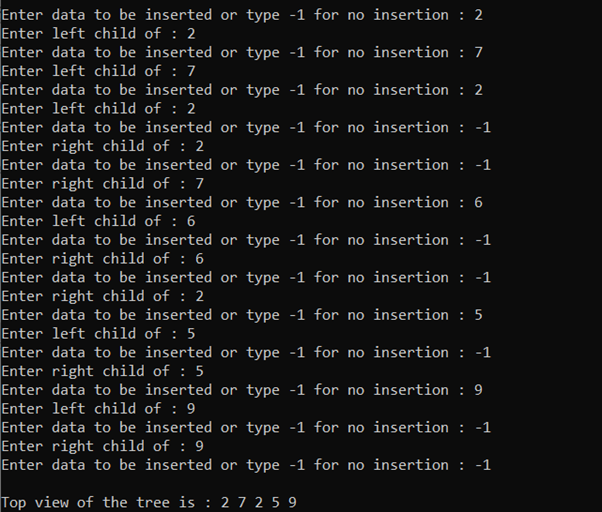Time Complexity: O(N)
Auxiliary Space: O(N)

### Bottom view of the tree:

Below is the program to illustrate the same:

## C++

 `// C++ program to demonstrate the``// above concepts``#include "bits/stdc++.h"``using` `namespace` `std;` `// Structure of the Binary Tree``struct` `treenode {``    ``int` `info;``    ``struct` `treenode *left,``        ``*right;``};` `// Function to create the Binary Tree``struct` `treenode* create()``{``    ``int` `data;``    ``struct` `treenode* tree;` `    ``// Dynamically allocating memory``    ``// for the tree-node``    ``tree = ``new` `treenode;` `    ``cout << ``"\nEnter data to be inserted "``         ``<< ``"or type -1 for no insertion : "``;` `    ``// Input from the user``    ``cin >> data;` `    ``// Termination Condition``    ``if` `(data == -1)``        ``return` `0;` `    ``// Assign value from user into tree``    ``tree->info = data;` `    ``// Recursively Call to create the``    ``// left and the right sub tree``    ``cout << ``"Enter left child of : "``         ``<< data;``    ``tree->left = create();` `    ``cout << ``"Enter right child of : "``         ``<< data;``    ``tree->right = create();` `    ``// Return the created Tree``    ``return` `tree;``};` `// Initialize an ordered Map``map<``int``, pair<``int``, ``int``> > HashMap;` `// Iterator for the map``map<``int``, pair<``int``, ``int``> >::iterator it;` `// Function to print the bottom view``// of the given binary tree``void` `bottomview(``struct` `treenode* root,``                ``int` `level, ``int` `height)``{``    ``// If root is NULL``    ``if` `(root == NULL)``        ``return``;` `    ``// If the height of the level is``    ``// greater than the current``    ``// stored height of the level``    ``if` `(height >= HashMap[level].second) {``        ``HashMap[level] = { root->info,``                           ``height };``    ``}` `    ``// Left and right recursive calls``    ``bottomview(root->left, level - 1,``               ``height + 1);``    ``bottomview(root->right, level + 1,``               ``height + 1);` `    ``return``;``}` `// Driver Code``int` `main()``{``    ``// Root Node``    ``struct` `treenode* root = NULL;` `    ``// Create the tree``    ``root = create();` `    ``bottomview(root, 0, 0);``    ``cout << ``"\nBottom view of the tree is : "``;` `    ``for` `(it = HashMap.begin();``         ``it != HashMap.end(); it++) {` `        ``cout << it->second.first << ``" "``;``    ``}` `    ``return` `0;``}` `/* Will be creating tree:``                ``2``           ``/     \``          ``7       5``         ``/  \       \``        ``2    6       9`` ``*/`

Output: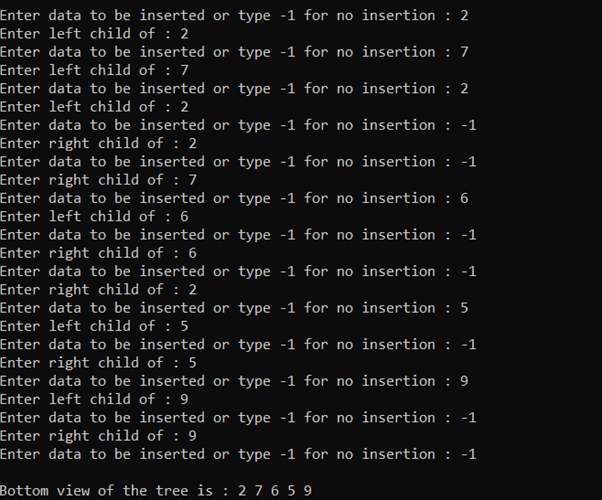Time Complexity: O(N)
Auxiliary Space: O(N)

### The mirror image of the tree:

Below is the program to illustrate the same:

## C++

 `// C++ program to implement``// the above approach``#include ``using` `namespace` `std;` `// structure of the binary tree``struct` `treenode {``    ``// data part``    ``int` `info;` `    ``// left and right node``    ``struct` `treenode *left, *right;``};` `// create function for binary``// tree creation``struct` `treenode* create()``{``    ``int` `data;` `    ``// variable of the structure``    ``struct` `treenode* tree;` `    ``// dynamically allocating``    ``// memory for tree-node``    ``tree = ``new` `treenode;` `    ``cout << ``"\nEnter data to be inserted or type -1 for no insertion : "``;` `    ``// input from the user``    ``cin >> data;` `    ``// condition for termination``    ``if` `(data == -1)``        ``return` `0;` `    ``// assigning value from user``    ``// into tree.``    ``tree->info = data;` `    ``// recursively calling create function``    ``// for left and right sub tree``    ``cout << ``"Enter left child of : "` `<< data;``    ``tree->left = create();` `    ``cout << ``"Enter right child of : "` `<< data;``    ``tree->right = create();` `    ``// returning the created tree``    ``return` `tree;``};` `/*``With the simple logic of recursion and``swapping, we can create mirror tree.``We will swap the left-node and``right-node of root node. We will use``recursion and start swapping from the``bottom of the tree.``*/` `// function to form mirror image a tree``void` `mirrortree(``struct` `treenode* root)``{``    ``if` `(root != NULL) {``        ``mirrortree(root->left);``        ``mirrortree(root->right);` `        ``struct` `treenode* temp;` `        ``temp = root->left;``        ``root->left = root->right;``        ``root->right = temp;``    ``}``    ``return``;``}` `// function for the inorder traversal``void` `inorder(``struct` `treenode* root)``{``    ``if` `(root == NULL)``        ``return``;` `    ``inorder(root->left);``    ``cout << root->info << ``"  "``;``    ``inorder(root->right);``}` `// Driver code``int` `main()``{``    ``// creating variable of the``    ``// structure``    ``struct` `treenode* root = NULL;` `    ``// calling create function to``    ``// create tree``    ``root = create();` `    ``mirrortree(root);``    ``cout << ``"\nInorder of the mirror tree is = "``;``    ``inorder(root);` `    ``return` `0;``}` `/* Will be creating tree:``                ``2``           ``/     \``          ``7       5``         ``/  \       \``        ``2    6       9`` ``*/`

Output: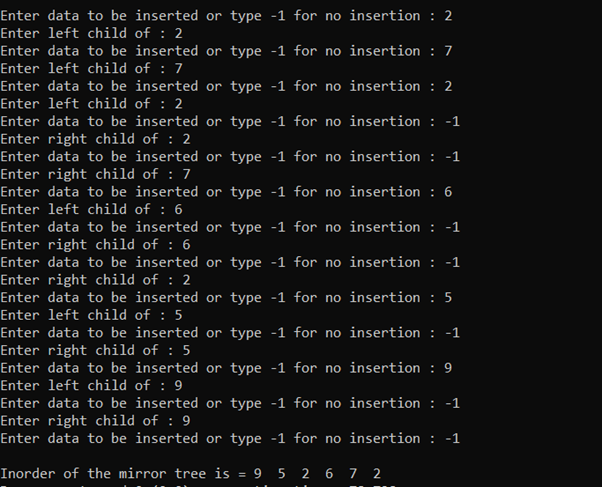Time Complexity: O(N)
Auxiliary Space: O(1)

### Serialize a tree:

Serialization of a tree is defined as the conversion of the given tree into a data-format that can be later restored and the structure of the tree must be maintained. Below is the program to implement the above approach:

## C++

 `// C++ program to implement``// the above approach``#include ``using` `namespace` `std;` `// structure of the binary tree``struct` `treenode {``    ``// data part``    ``int` `info;` `    ``// left and right node``    ``struct` `treenode *left, *right;``};` `// create function for binary``// tree creation``struct` `treenode* create()``{``    ``int` `data;` `    ``// variable of the structure``    ``struct` `treenode* tree;` `    ``// dynamically allocating``    ``// memory for tree-node``    ``tree = ``new` `treenode;` `    ``cout << ``"\nEnter data to be inserted or type -1 for no insertion : "``;` `    ``// input from the user``    ``cin >> data;` `    ``// condition for termination``    ``if` `(data == -1)``        ``return` `0;` `    ``// assigning value from user``    ``// into tree.``    ``tree->info = data;` `    ``// recursively calling create function``    ``// for left and right sub tree``    ``cout << ``"Enter left child of : "` `<< data;``    ``tree->left = create();` `    ``cout << ``"Enter right child of : "` `<< data;``    ``tree->right = create();` `    ``// returning the created tree``    ``return` `tree;``};` `// Function to serialize the given``// Binary Tree``void` `serialize(``struct` `treenode* root,``               ``vector<``int``>& v)``{``    ``// If the root is NULL, then``    ``// push -1 and return``    ``if` `(root == NULL) {``        ``v.push_back(-1);``        ``return``;``    ``}` `    ``// Otherwise, push the data part``    ``v.push_back(root->info);` `    ``// Recursively Call for the left``    ``// and the right Subtree``    ``serialize(root->left, v);``    ``serialize(root->right, v);``}` `// Driver Code``int` `main()``{``    ``// Root Node``    ``struct` `treenode* root = NULL;` `    ``// Create a tree``    ``root = create();` `    ``vector<``int``> v;` `    ``serialize(root, v);``    ``cout << ``"\nSerialize form of the tree is = "``;` `    ``for` `(``int` `i = 0; i < v.size(); i++)``        ``cout << v[i] << ``"  "``;``    ``return` `0;``}` `/* Will be creating tree:``                ``2``           ``/     \``          ``7       5``         ``/  \       \``        ``2    6       9`` ``*/`

## Java

 `import` `java.util.*;` `// structure of the binary tree``class` `TreeNode {``    ``// data part``    ``int` `info;` `    ``// left and right node``    ``TreeNode left, right;` `    ``// constructor``    ``TreeNode(``int` `item)``    ``{``        ``info = item;``        ``left = right = ``null``;``    ``}``}` `class` `SerializeDeserializeBinaryTree {``    ``// create function for binary``    ``// tree creation``    ``public` `static` `TreeNode create()``    ``{``        ``int` `data;` `        ``// variable of the structure``        ``TreeNode tree;` `        ``// dynamically allocating``        ``// memory for tree-node``        ``tree = ``new` `TreeNode(``0``);` `        ``Scanner sc = ``new` `Scanner(System.in);``        ``System.out.print(``            ``"\nEnter data to be inserted or type -1 for no insertion : "``);` `        ``// input from the user``        ``data = sc.nextInt();` `        ``// condition for termination``        ``if` `(data == -``1``)``            ``return` `null``;` `        ``// assigning value from user``        ``// into tree.``        ``tree.info = data;` `        ``// recursively calling create function``        ``// for left and right sub tree``        ``System.out.print(``"Enter left child of : "` `+ data);``        ``tree.left = create();` `        ``System.out.print(``"Enter right child of : "` `+ data);``        ``tree.right = create();` `        ``// returning the created tree``        ``return` `tree;``    ``}` `    ``// Function to serialize the given``    ``// Binary Tree``    ``public` `static` `void` `serialize(TreeNode root,``                                 ``List v)``    ``{``        ``// If the root is NULL, then``        ``// push -1 and return``        ``if` `(root == ``null``) {``            ``v.add(-``1``);``            ``return``;``        ``}` `        ``// Otherwise, push the data part``        ``v.add(root.info);` `        ``// Recursively Call for the left``        ``// and the right Subtree``        ``serialize(root.left, v);``        ``serialize(root.right, v);``    ``}` `    ``// Driver Code``    ``public` `static` `void` `main(String args[])``    ``{``        ``// Root Node``        ``TreeNode root = ``null``;` `        ``// Create a tree``        ``root = create();` `        ``List v = ``new` `ArrayList();` `        ``serialize(root, v);``        ``System.out.print(``            ``"\nSerialize form of the tree is = "``);` `        ``for` `(``int` `i = ``0``; i < v.size(); i++)``            ``System.out.print(v.get(i) + ``"  "``);``    ``}``}` `/* Will be creating tree:``2``/``7 5``/ \``2 6 9``*/`

Output: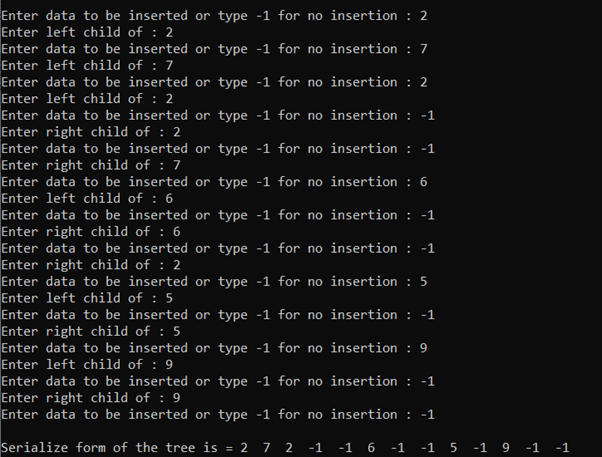Time Complexity: O(N)
Auxiliary Space: O(N)

Complexity Analysis:

1. Time Complexity: O(n).
2. Auxiliary Space: O(1).

My Personal Notes arrow_drop_up